## Example Questions

### Example Question #7 : Polynomial Operations

Expand: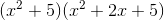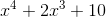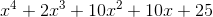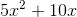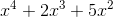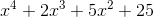Explanation:

Expand:Step 1: Use the distributive property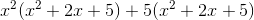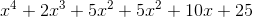Step 2: Combine like terms### Example Question #8 : Polynomial Operations

Two positive consecutive whole numbers that are even are both multiples of. The product of the two numbers is. What is the sum of the two integers?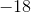Explanation:

The question provides two positive whole numbers that are each multiples of 6 and also 6 numbers apart. This may be translated into variables, where the first number may be represented by "x" and the second number may be represented as "x+6" given that it is 6 numbers greater than x.

The problem provides the information that the product of these two numbers is 72. Using the new definitions for the numbers, this may be represented as:

(x)(x+6) = 72

This provides an equation that multiplies two polynomials (one with one term, which is a monomial and one with two terms, which is a binomial) and an ability to solve for what x (the first of the two numbers) may be.

Using FOIL, the result is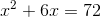. This may be rewritten as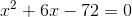, which will provide the value of x after factoring.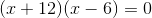, where (-6)(12) will provide the product of -72 and the sum of 12 and -6 will yield 6. The results indicate x as having two possible solutions: x=6 and x=-12. Returning back to the question, the goal is to find two positive numbers. This means that x=-12 is not a viable solution and that x=6 is. Now, revisiting the terms used to redefine the two numbers [x and x+6], x has been calculated. After substituting in the x value for the second term, the second number is 12 (6+6=12).

The final step of the problem is to solve for the sum of these two numbers:
6+12=18

### Example Question #9 : Polynomial Operations

Multiply these polynomials out and expand.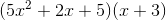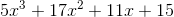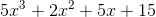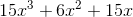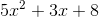Explanation:

The proper way to multiply polynomials is to essentially apply the distributive property continually.

Sois really the same as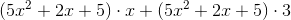, and so on.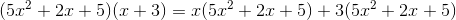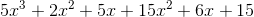Then, simply group together like terms to get the final answer: